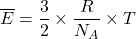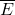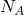## If energy is going into a system, but not being converted into kinetic energy, it will be converted into potential energy. This will be repr

Question

If energy is going into a system, but not being converted into kinetic energy, it will be converted into potential energy. This will be represented by a __________________ on a heating curve, which occur at the __________________ and ____________________.

in progress 0
2 months 2021-07-30T18:20:17+00:00 1 Answers 0 views 0

1. Plateau where the temperature stays constant

2. Melting

3. Boiling points of the substance

Explanation:

The relationship between kinetic energy and temperature is given as follows;Where;= The average kinetic energy of the atom

R = The universal gas constant= Avogadro’s number

T = The temperature in Kelvin

Therefore, the kinetic energy increases as the temperature increases, however when the energy is not being converted into kinetic energy, the energy is stored as internal potential energy in the melted or evaporated state of the substance

Therefore, we have;

If energy is going into a system, but not being converted into kinetic energy, it will be converted into potential energy. This will be represented by a 1. plateau where the temperature stays constant on a heating curve, which occur at the 2. melting and 3. boiling points of the substance.